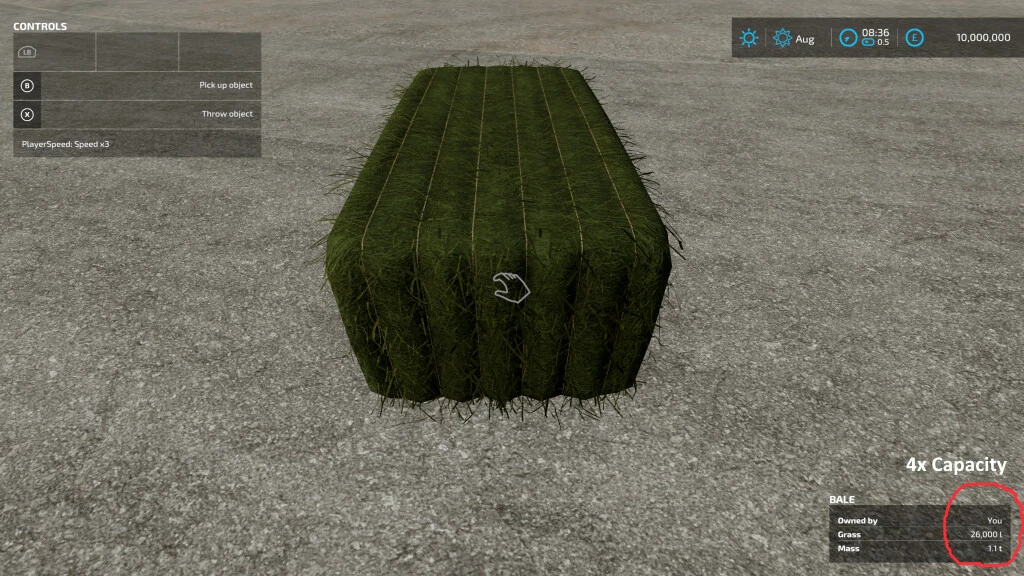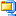This pack includes modified versions of all the base game Balers that now allow you to select a bale capacity of 2x, 3x & 4x the standard bale capacity.
Also included are modified base game Bale Loaders & Bale Wrappers that support the larger capacity bales.
The increased capacities are displayed in the CONTROLS window of the HUD as additional bale size options and can be selected as you would normally select bale sizes.

The size options displayed are:
120cm = standard. 122 = 2x, 123 = 3x, 124 = 4x
125cm = standard. 132 = 2x, 133 = 3x, 134 = 4x
150cm = standard. 152 = 2x, 153 = 3x, 154 = 4x
180cm = standard. 182 = 2x, 183 = 3x, 184 = 4x
220cm = standard. 222 = 2x, 223 = 3x, 224 = 4x
240cm = standard. 242 = 2x, 243 = 3x, 244 = 4x

NOTE: Due to the modified bale sizes any Bale Loaders & Bale Wrappers that are NOT modified to support the new sizes will not work.

Credits:

GH66Mods

File File sizeFS22_SelectableBaleCapacity 194 KB

#### You may also like...

Subscribe
Notify of